# NCERT Solutions For Class 10 Maths Chapter 8 Exercise 8.4 – Introduction to Trignometry

Download NCERT Solutions For Class 10 Maths Chapter 8 Exercise 8.4 – Introduction to Trignometry. This Exercise contains 5 questions, for which detailed answers have been provided in this note. In case you are looking at studying the remaining Exercise for Class 10 for Maths NCERT solutions for other Chapters, you can click the link at the end of this Note.

### NCERT Solutions For Class 10 Maths Chapter 8 Exercise 8.4 – Introduction to Trignometry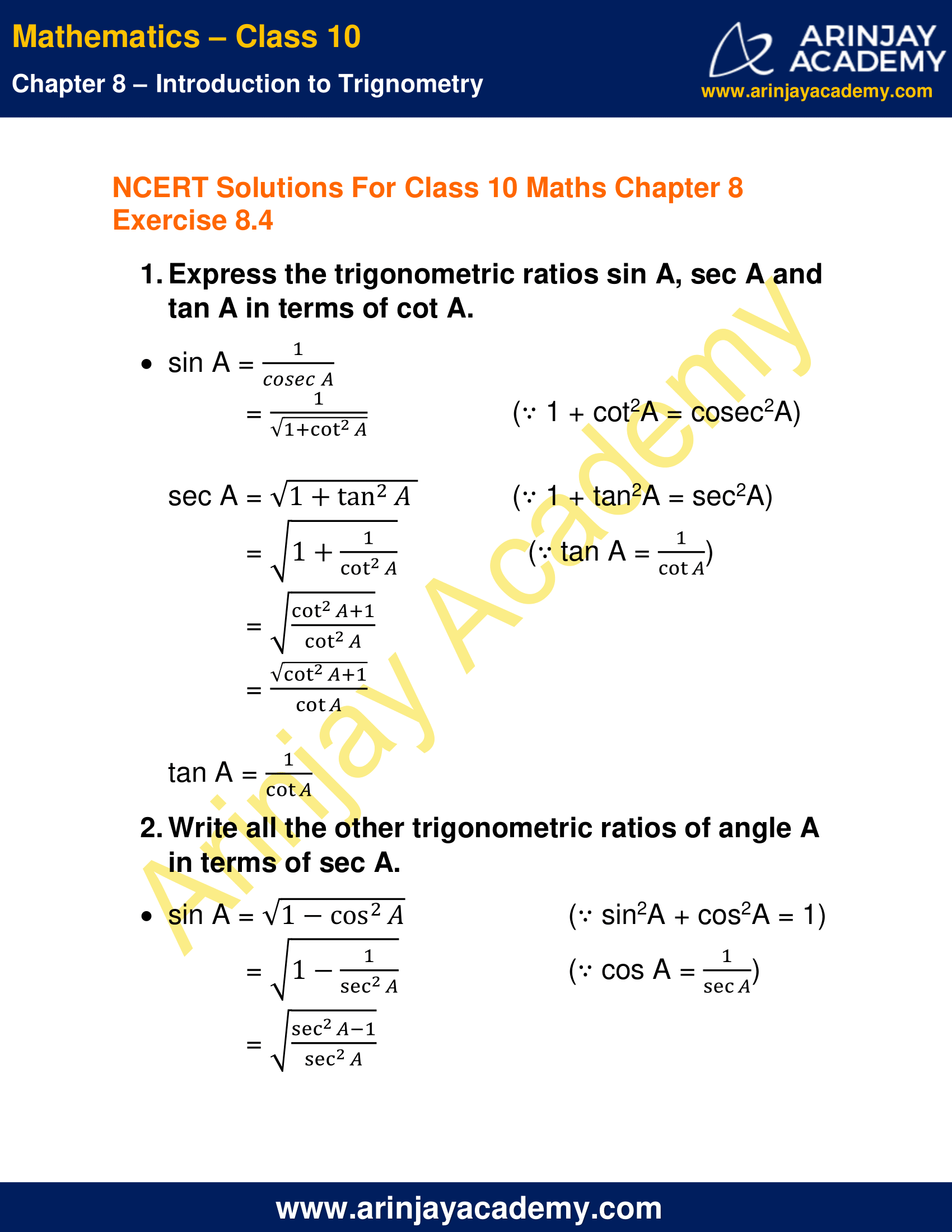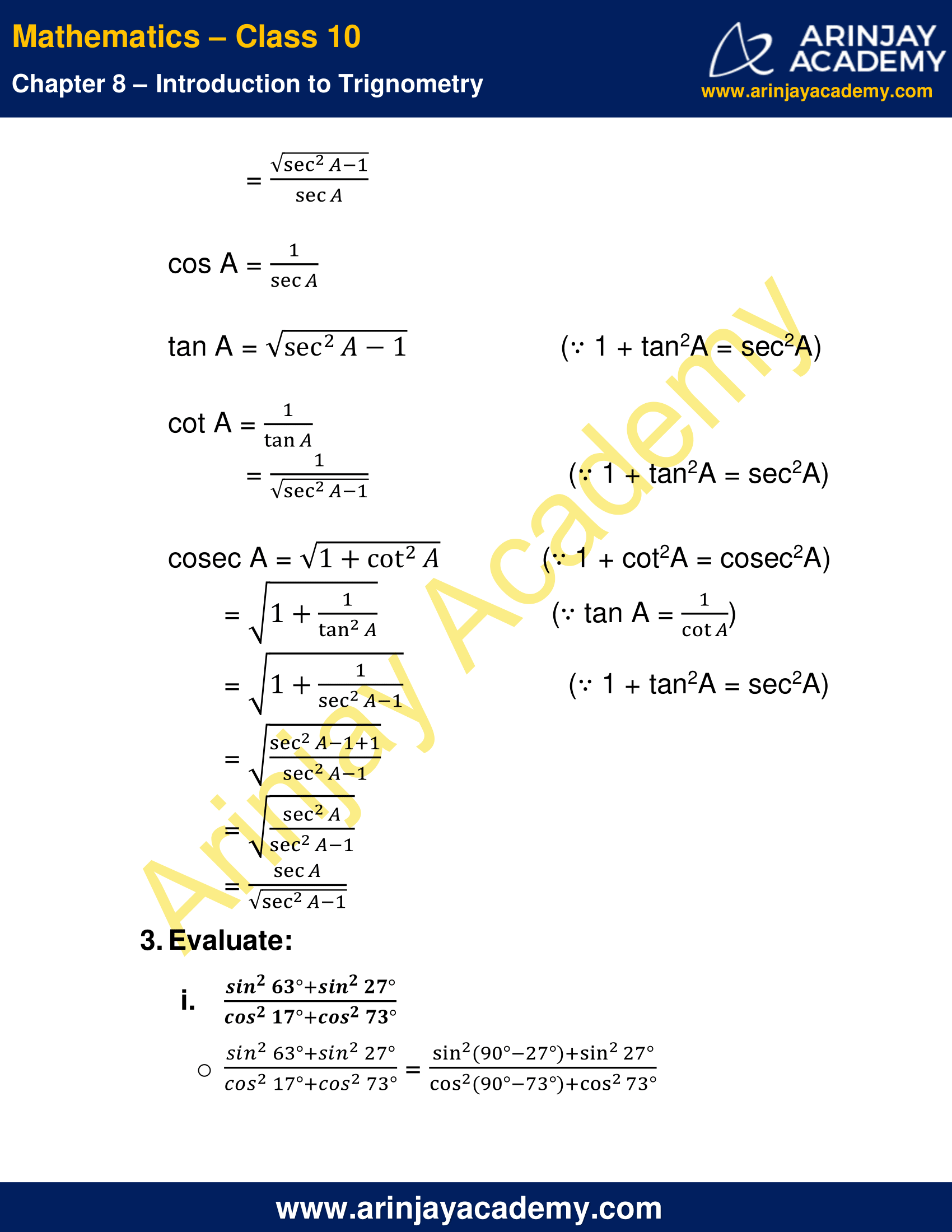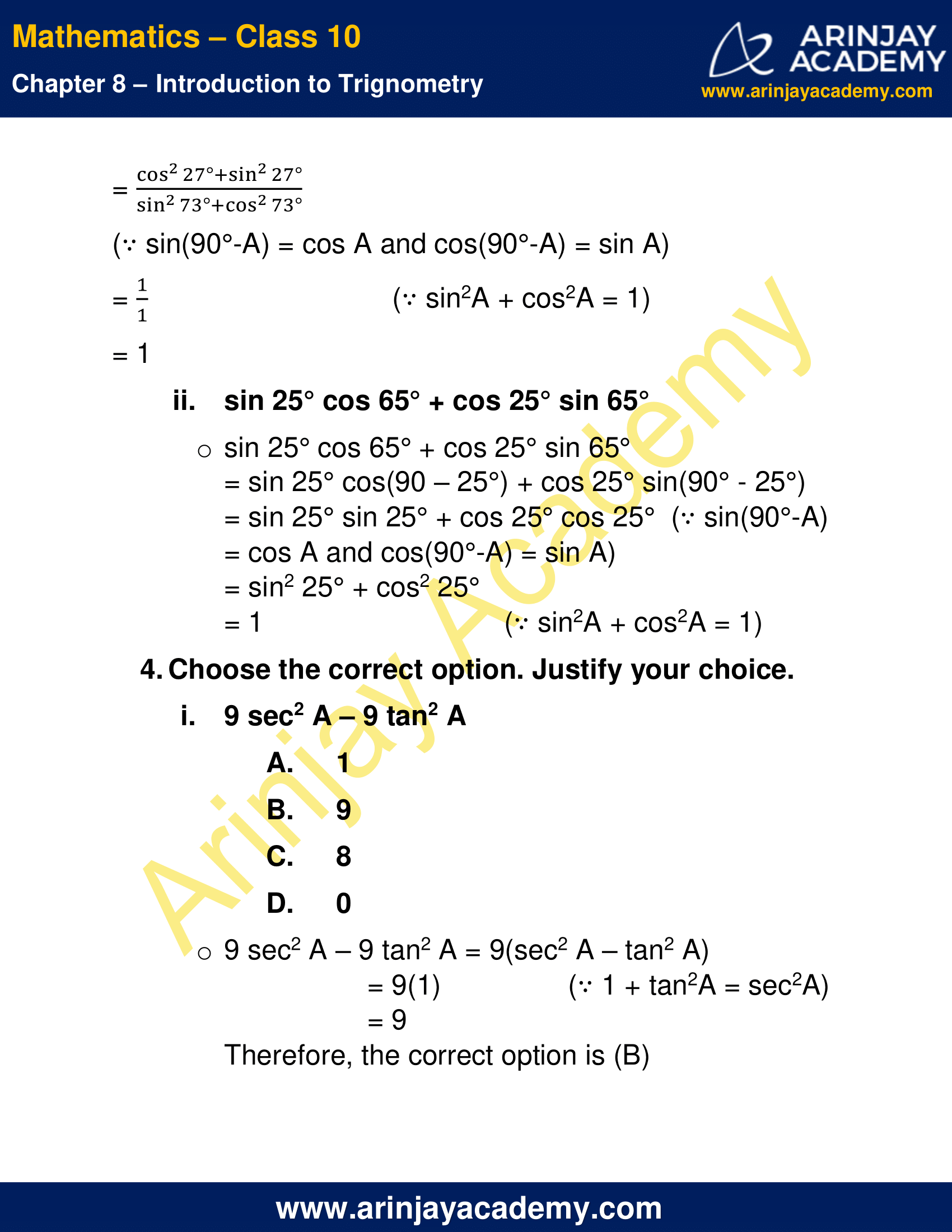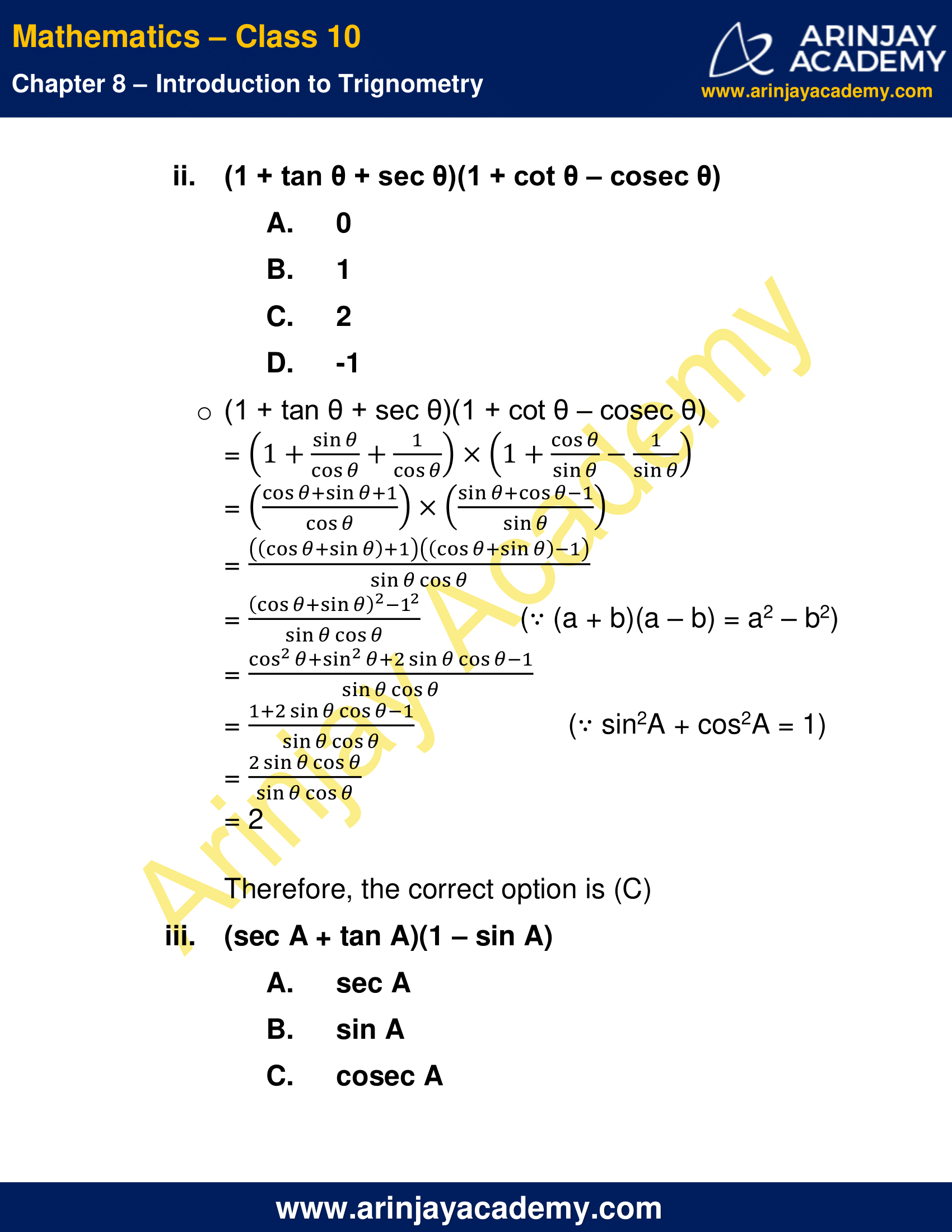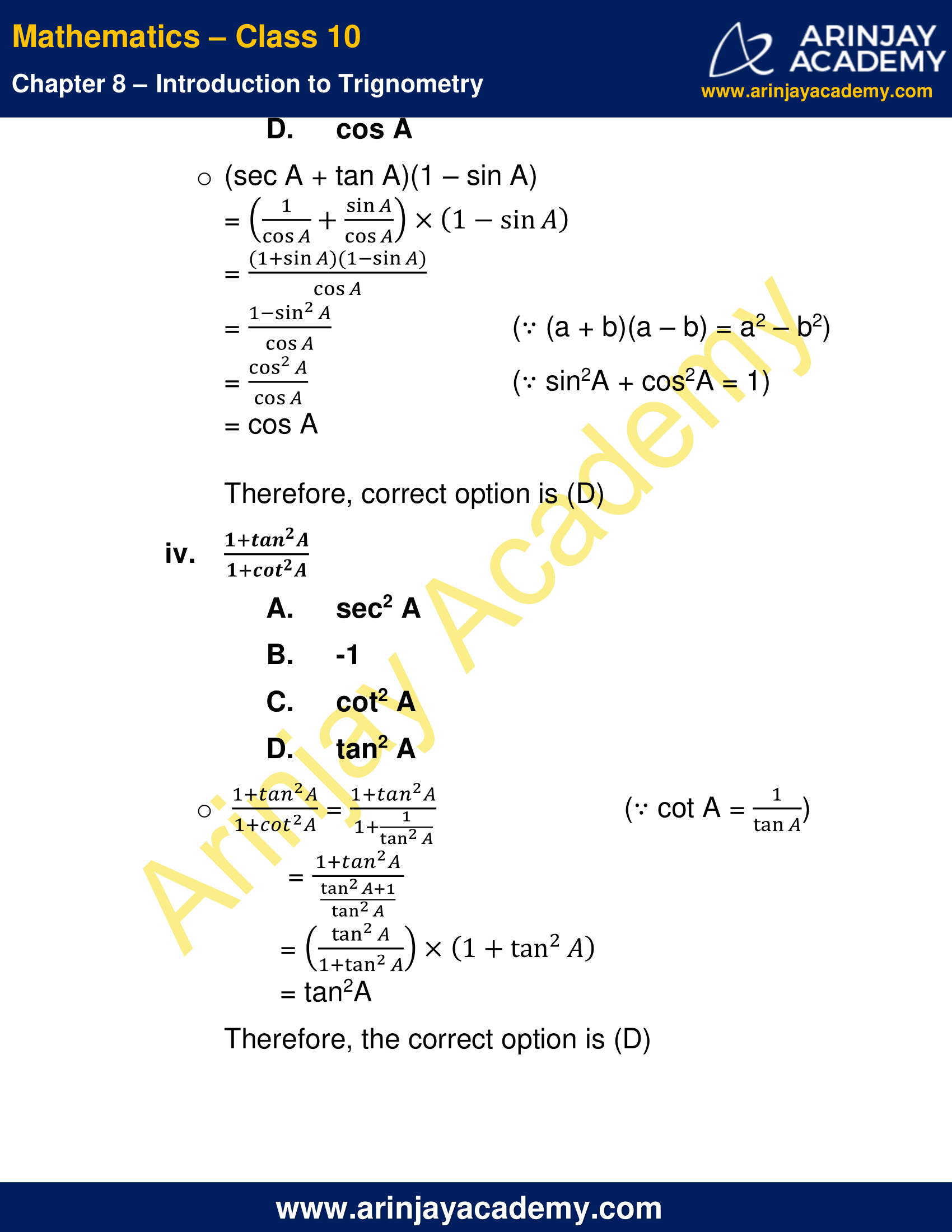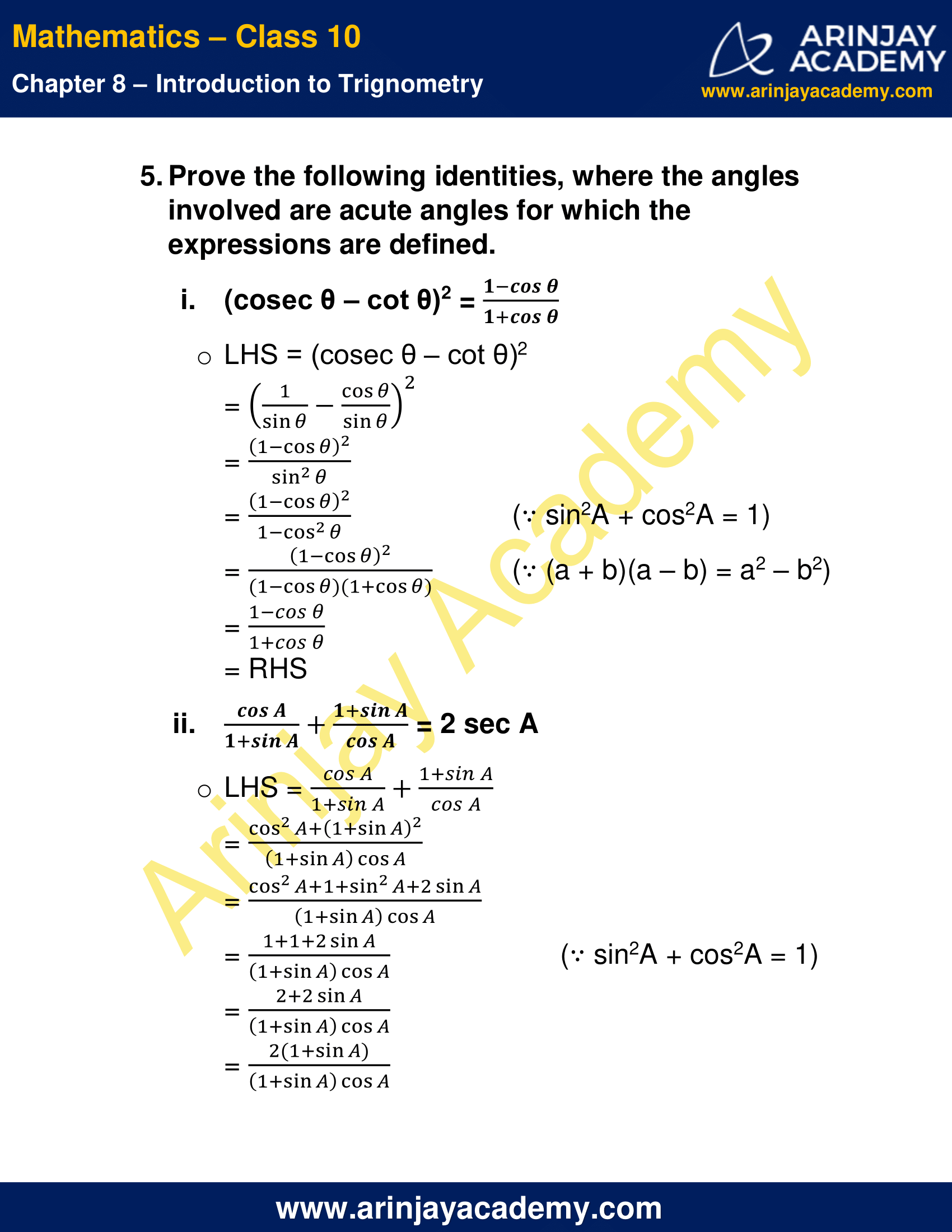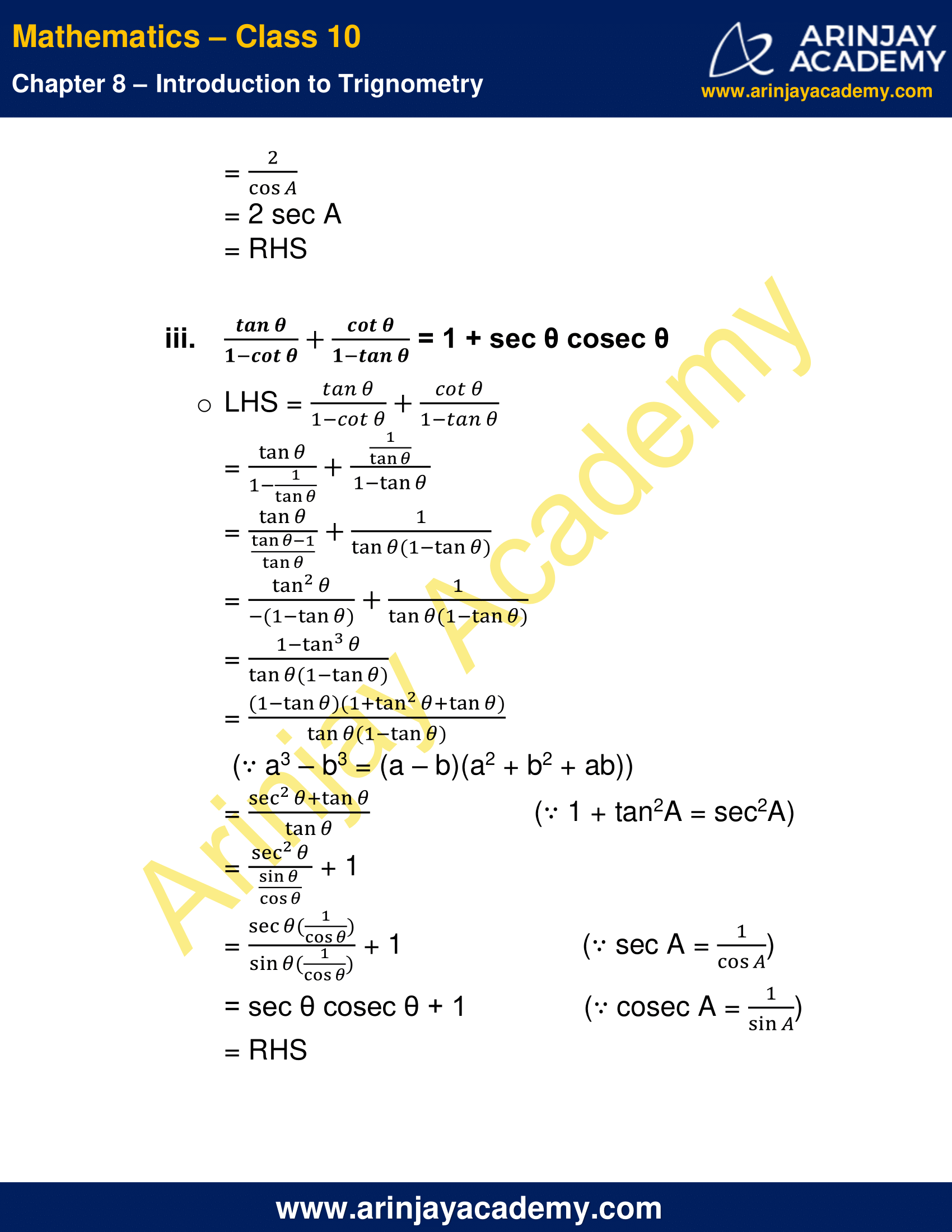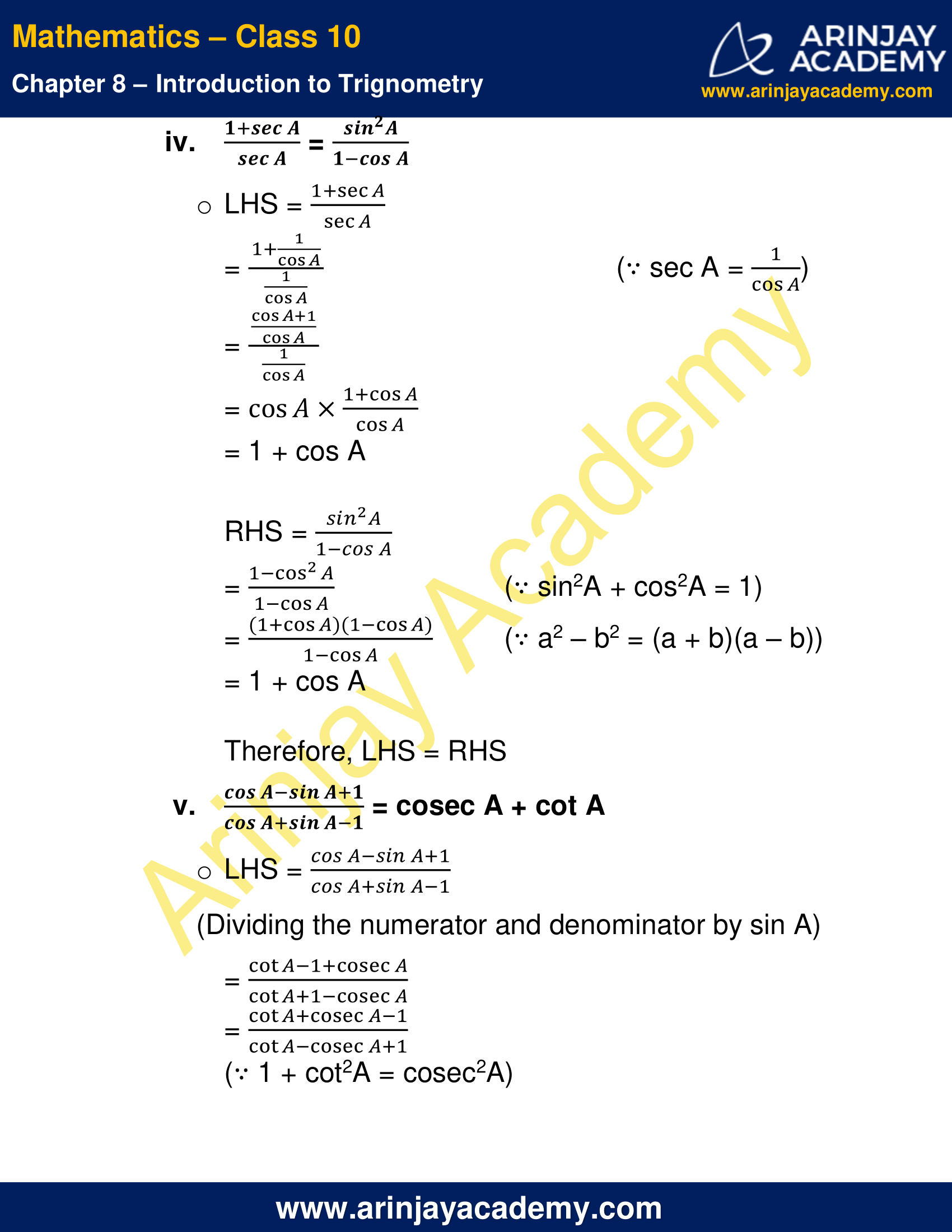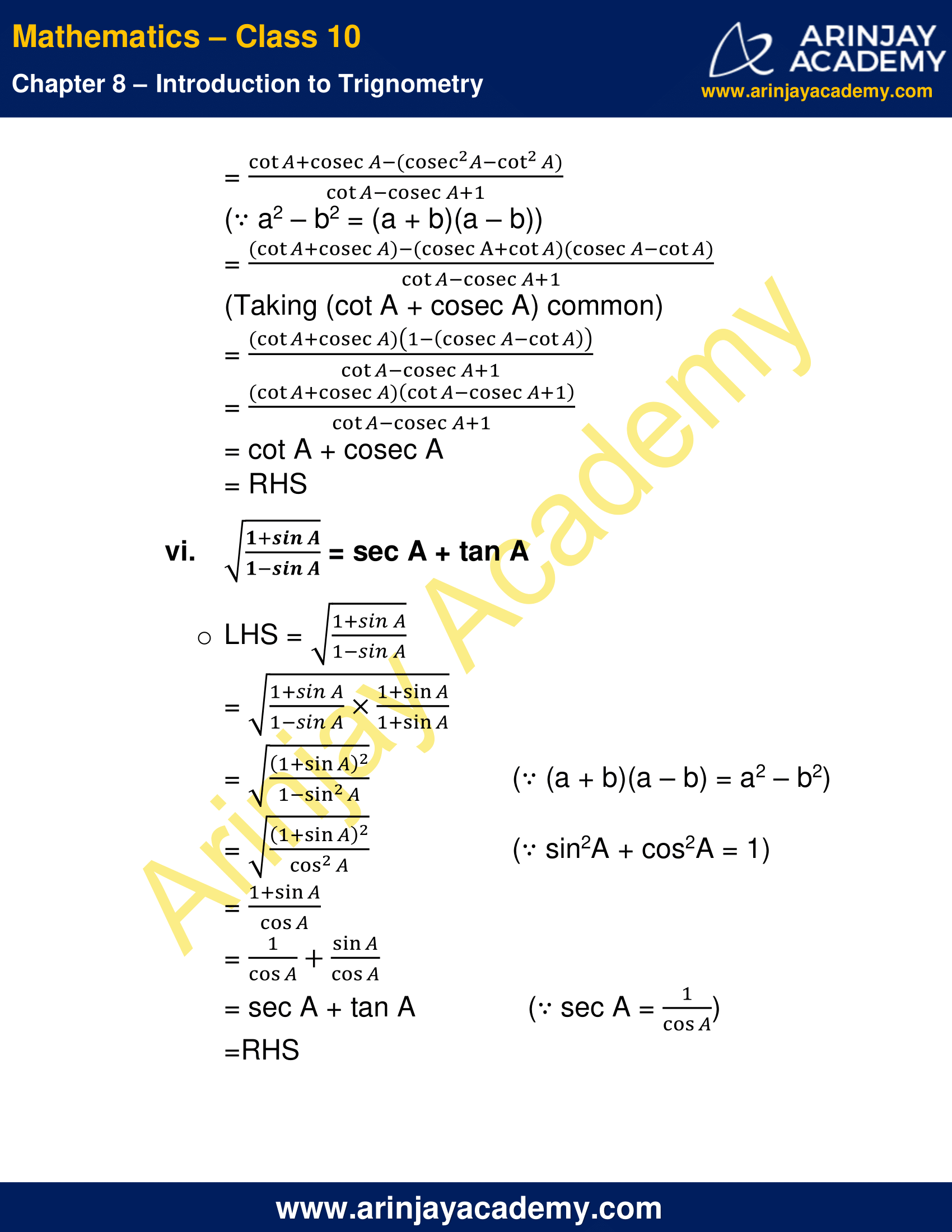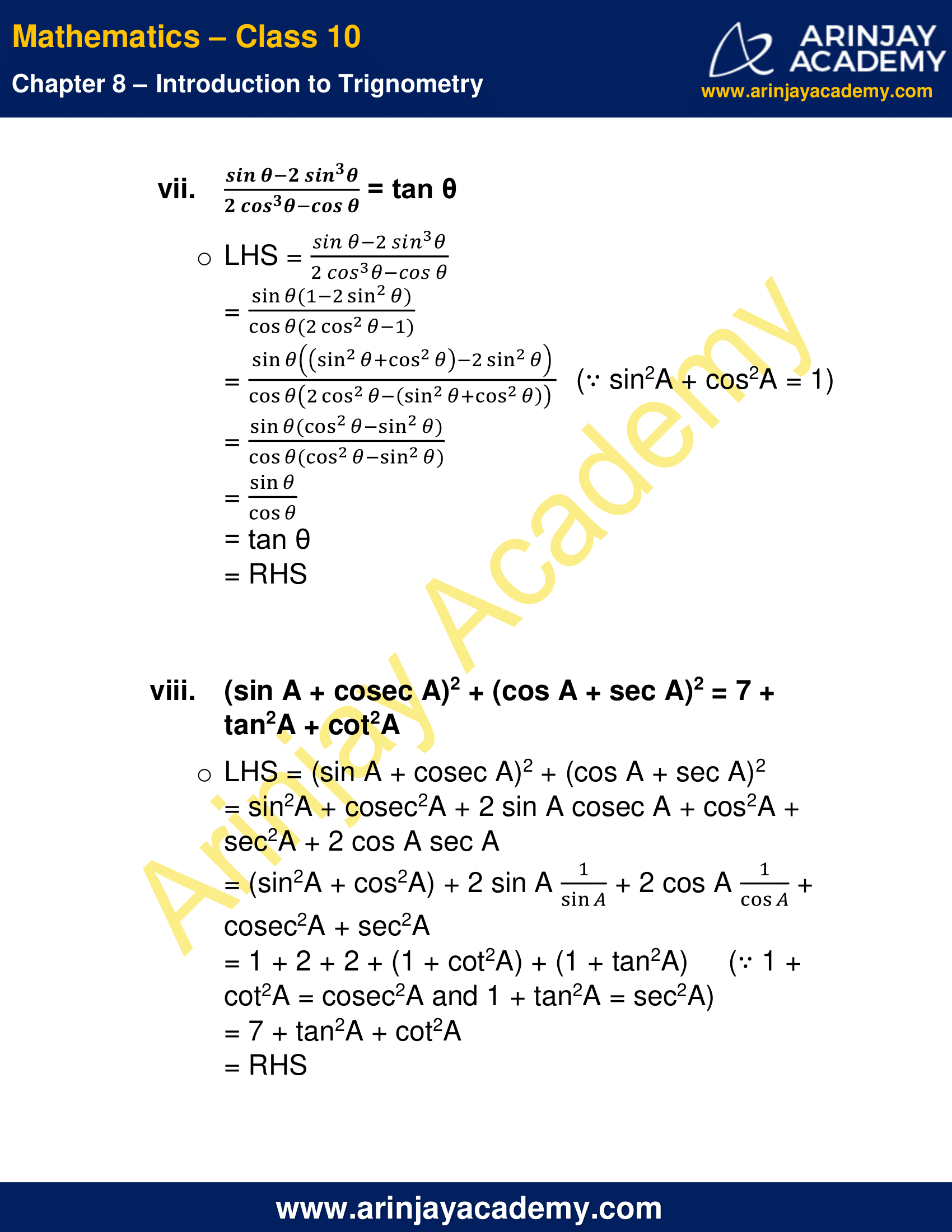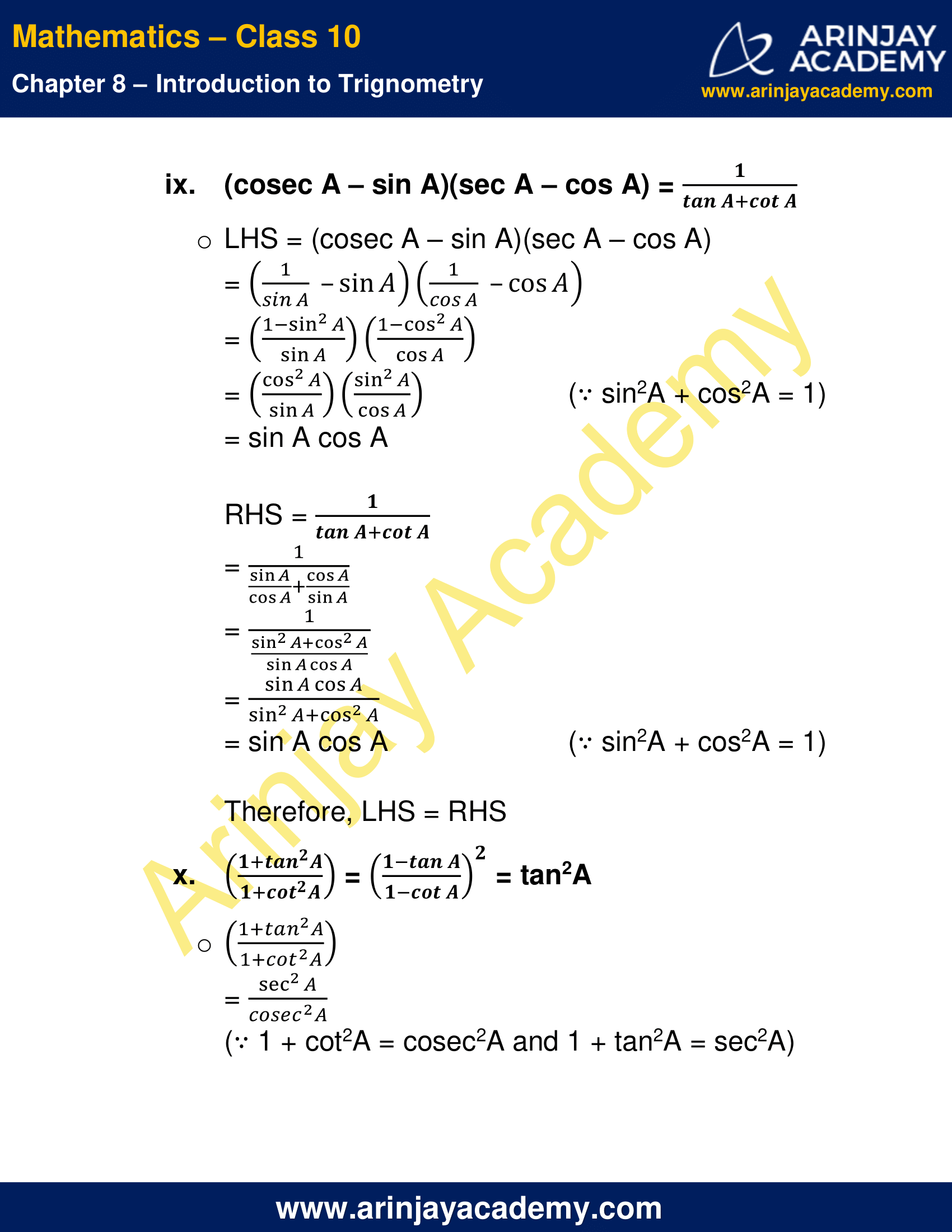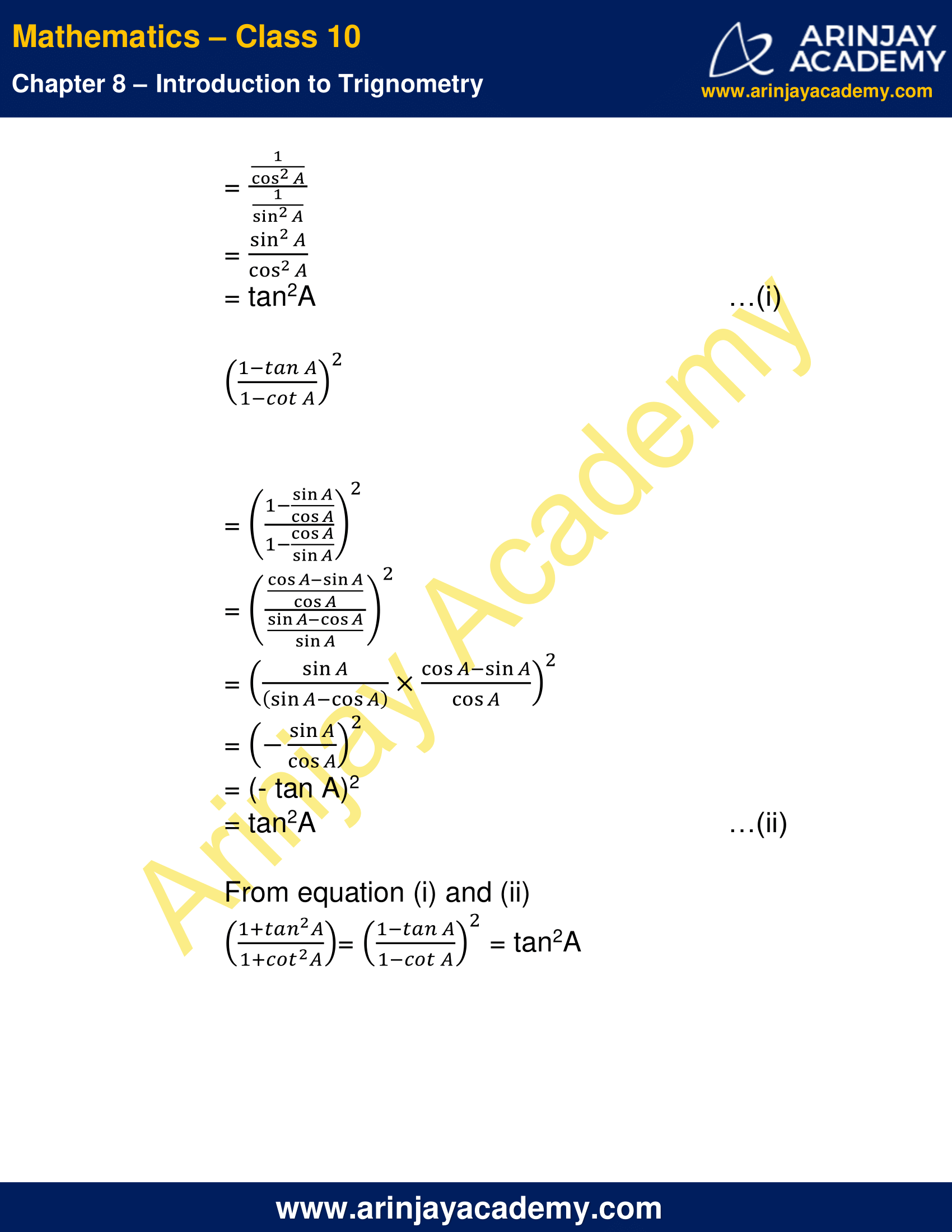NCERT Solutions for Class 10 Maths Chapter 8 Exercise 8.4 – Introduction to Trignometry, has been designed by the NCERT to test the knowledge of the student on the topic – Trigonometric Identities

### 3 thoughts on “NCERT Solutions For Class 10 Maths Chapter 8 Exercise 8.4 – Introduction to Trignometry”

1.This was so amazing and more helpful to me
But say plzz how to down load PDF

•2.